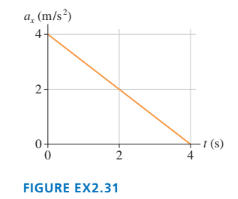# Problem: FIGURE EX2.31 shows the acceleration-versus-time graph of a particle moving along the x-axis. Its initial velocity is v0x = 8.0 m/s at t 0 = 0 s. What is the particle’s velocity at t = 4.0 s?

🤓 Based on our data, we think this question is relevant for Professor Navarro Perez's class at SDSU.

###### Problem Details

FIGURE EX2.31 shows the acceleration-versus-time graph of a particle moving along the x-axis. Its initial velocity is v0x = 8.0 m/s at t 0 = 0 s. What is the particle’s velocity at t = 4.0 s?